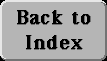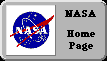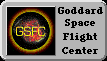# (15) Energy

## Potential and KineticAn interesting thing about the final speed of an object descending (with no friction) from some given height h, along a sloping surface: one can change the slope, one can even change the shape of the surface--yet the final speed v with which it reaches the bottom will always be the same. If it were not for friction, any skier gliding down a snowy hill from the summit to the base should arrive with the same speed, whether the path taken is the easy beginner's slope or the steep experts-only route.

Reducing the slope of the surface reduces the acceleration a, but it also lengthens the time of descent, and these two variations cancel, leaving the final speed unchanged. The same speed is also obtained if the object falls vertically from that height h, and in that case it is easily derived, as follows. The duration t of the fall is given by

h = g t2/ 2

Multiply both sides by g:

gh = g2t2/ 2

Then since the final velocity is

v = gt

one gets

gh = v2/ 2

By the last equation, as the object loses elevation--assuming nothing interferes with its motion--v2 grows, and as noted, this growth does not depend on the path taken.

This exchange between h and v2 also works in the opposite direction: an object rolling up an incline loses v2 in direct proportion to the elevation h it gains. A marble rolling down the inside of a smooth bowl gathers speed as it approaches the bottom, then as it shoots up the other side it loses all of it again. If no friction existed, it would rise again to the same height as the one from which it had started.

A simple pendulum, or a child on a swing, also trades height for v2and back again, in the same manner. And bicycle riders are well aware that the speed gained rolling down a hillside can be traded for height when climbing the next slope. It is as if height gave us something with which we could purchase speed, and which later, if the occasion demanded, could be converted back to height.

That "something" is called energy. It was already briefly discussed in an earlier section.

This back and forth trading suggests that perhaps the sum

gh + v2/ 2

has a constant value: if one part decreases, the other part must get bigger. Is that sum the energy? Not quite. The effort of getting heavy load up a height h is greater than that of lifting a light one. Let us now call the amount of matter in an object its "mass. " It is obviously proportional to the object's weight, but as will later be seen, the concept of mass is more complicated than that.

If energy is to measure the effort in lifting a load, it should also be proportional to its mass m. We thus multiply everything by m and write

Energy = E = mgh + mv2/ 2A well-known fact--already hinted at--is that in a system which does not interact with its surroundings, the total energy (denoted here by the letter E) stays the same ("is conserved"). In a pendulum at the extreme point of its swing, v = 0 and therefore the second term above vanishes, while the first term is at its biggest. Then as the mass descends descends, mv2/ 2 increases and mgh drops, until at the bottom of the swing the first term is at its smallest and the second reaches maximum. On the upswing the process reverses, and the sequence is repeated for every swing.

Both terms in the equation above have names: mgh is the potential energy, the energy of position, and mv2/ 2 is the kinetic energy, the energy of motion.

The exact number representing E will obviously depend on where h is measured from (the floor? sea level? the center of the Earth? ). Different choices are possible, and each leads to a different value of E: the formula is thus meaningful only if a certain reference height is chosen where h=0.

## Other kinds of energy

Textbooks define energy as "the ability to do work" and they define work as "overcoming resistance over a distance". For instance, if m is the mass of a brick, the force on it is mg and lifting it against gravity to a height h, against the pull of gravity, requires the performance of work W, with

W = mgh

Dragging that brick a distance x along level ground against the force of friction F similarly requires the performance of work

W = Fx

For the record, work is measured in joules, after James Prescott Joule (1818-89), a brewer in Manchester, England, whose experiments helped establish the fact that heat was a form of energy (see further below) and not some mysterious fluid permeating matter. Since any such work can be performed by a machine, one can also loosely define energy as anything that can cause a machine to turn.

Devices or processes that convert energy
from one form (column) to another (row)
- Kinetic Potential Heat Light Chemical Electric
Kinetic ***** Pendulum Rocket Nozzle Solar sail Muscles Electric motor
Potential Pendulum ***** Steam boiler x x x
Heat Friction x ***** Solar heater Fire Electric stove
Light x x Lightbulb, Sun ***** Firefly light Light emitting diode
Chemical x x Quicklime kiln Green plants ***** Car battery
Electric Windmill power Hydroelectric power Thermocouple Solar Cell Flashlight battery *****

Energy is also measured in joules. In many ways it resembles money: it is a currency in which all processes in nature must be paid for. Just as money can come in dollars, pesos, yen, rubles or liras, so energy can come in many forms--electricity, heat, light, sound, chemical, nuclear. The expression for the total energy of a system of objects can be written

E = (potential) + (kinetic) + (electric) + (heat) + . . .

where "kinetic" for instance stands for the sum of mv2/2 for all the component parts. And it is still true that if the system does not interact with the outside, the total value of E is conserved.

It is also true that in most cases, with proper tools, one form of energy can be converted into another: light shining on solar cells generates electricity, which can turn the motor of a fan, providing the kinetic energy of rotating blades, or run a radio, producing sound.

## Units

Like currencies, different kinds of energy have a certain exchange rate: in the exchange between kinetic or potential energy and heat, for instance, one calorie equals about 4.18 joules. The chemical energy of food is also measured in calories, though one should note these are "big" calories or kilocalories, each equal to 1000 of the small kind.

The rate at which energy is supplied or used is called power and is measured in watts, after the inventor of the modern steam engine, the Scotsman James Watt (1736-1818): an energy supply providing one joule per second gives one watt of power. Thus a 60-watt bulb provides 60 joules each second--about as much as a biker pedaling up a hill. Bills for electric energy, sent out by power companies, are usually calculated at so many dollars, pesos, yen etc. per kilowatt-hour (kwh)--the energy of one kilowatt or 1000 watt, supplied for one hour. Since the hour has 3600 seconds, it follows that one kwh equals 3 600 000 joules.

## Heat

When a bank changes money from one currency to another, it usually charges some percentage as the cost of the transaction. The same happens in the exchange of energy from one form to another: you always get less than what you put in. The marble sliding down the side of the bowl, for instance, always rises on the other side to a lower elevation than the one it started from.

The missing energy is not lost, however, but ends up as heat. Heat is the "soft currency" of the energy universe: it is possible to convert heat to other forms of energy (in a steam engine, for instance), but one can never get the full value. That, essentially, is the "second law of thermodynamics," a fundamental law related to the nature of heat. The missing amount not only remains as heat, but becomes heat at a lower temperature, from which only an even smaller fraction can be converted into other forms. It would solve all of mankind's energy problems if, for instance, one could extract the heat energy of the oceans, leaving them slightly cooler and converting the extracted heat to electricity; but the second law tells that it cannot be done.

### Tidbits

Packaged foods in the US carry labels listing their nutrients and the amount of calories (actually, kilocalories) per portion and per given weight. In other countries the information may differ, and the British "Cadbury's Wispa" milk chocolate bar offers a choice of kilocalories and kilojoules:

 . Bar 100 gr. Energy Kj 885 2300 ..........Kcal 210 550 Protein 2.7 gr 7.1 Carbohyd. 20.8 53.9 Fat 13.2 34.2

Next Stop: #16 Newton and his LawsAuthor and curator: David P. Stern, u5dps@lepvax.gsfc.nasa.gov
Last updated 24 August 1998